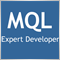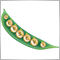How do i arrange or the IF ELSE Functions622

if(iClose(_Symbol,_Period,1)<MA50 && iOpen(_Symbol,_Period,1)<MA50 && iHigh(_Symbol,_Period,1)<MA50 && iLow(_Symbol,_Period,1)<MA50
&& iClose(_Symbol,_Period,1)<BBDn && MA5L>BBDn)
{mess_data[k][j]="TM";mess_color[k][j]=clrRed;}

else if(iClose(_Symbol,_Period,1)<MA50 && iOpen(_Symbol,_Period,1)<MA50 && iHigh(_Symbol,_Period,1)<MA50 && iLow(_Symbol,_Period,1)<MA50)
{mess_data[k][j]="T";mess_color[k][j]=clrRed;}

else if(iClose(_Symbol,_Period,1)<BBDn && MA5L>BBDn)
{mess_data[k][j]="M";mess_color[k][j]=clrRed;}

if(iClose(_Symbol,_Period,1)>MA50 && iOpen(_Symbol,_Period,1)>MA50 && iHigh(_Symbol,_Period,1)>MA50 && iLow(_Symbol,_Period,1)>MA50
&& iClose(_Symbol,_Period,1)>BBUp && MA5H<BBUp)
{mess_data[k][j]="TM";mess_color[k][j]=clrBlue;}

else if(iClose(_Symbol,_Period,1)>MA50 && iOpen(_Symbol,_Period,1)>MA50 && iHigh(_Symbol,_Period,1)>MA50 && iLow(_Symbol,_Period,1)>MA50)
{mess_data[k][j]="T";mess_color[k][j]=clrBlue;}

else if(iClose(_Symbol,_Period,1)>BBUp && MA5H<BBUp)
{mess_data[k][j]="M";mess_color[k][j]=clrBlue;}

else {mess_data[k][j]="X";mess_color[k][j]=clrGray;}

Hi, top here is the code. cause i have much if, it didn't works well. need someone to help me

if(iClose(_Symbol,_Period,1)<MA50 && iOpen(_Symbol,_Period,1)<MA50 && iHigh(_Symbol,_Period,1)<MA50 && iLow(_Symbol,_Period,1)<MA50
&& iClose(_Symbol,_Period,1)<BBDn && MA5L>BBDn)
{mess_data[k][j]="TM";mess_color[k][j]=clrRed;}

else if(iClose(_Symbol,_Period,1)<MA50 && iOpen(_Symbol,_Period,1)<MA50 && iHigh(_Symbol,_Period,1)<MA50 && iLow(_Symbol,_Period,1)<MA50)
{mess_data[k][j]="T";mess_color[k][j]=clrRed;}

else if(iClose(_Symbol,_Period,1)<BBDn && MA5L>BBDn)
{mess_data[k][j]="M";mess_color[k][j]=clrRed;}

this one group of signal. this for my dashboard. The first if is the main among the other 2. if it's false then it proceed to next if. if it's false then it will proceed to next if.

same goes for

if(iClose(_Symbol,_Period,1)>MA50 && iOpen(_Symbol,_Period,1)>MA50 && iHigh(_Symbol,_Period,1)>MA50 && iLow(_Symbol,_Period,1)>MA50
&& iClose(_Symbol,_Period,1)>BBUp && MA5H<BBUp)
{mess_data[k][j]="TM";mess_color[k][j]=clrBlue;}

else if(iClose(_Symbol,_Period,1)>MA50 && iOpen(_Symbol,_Period,1)>MA50 && iHigh(_Symbol,_Period,1)>MA50 && iLow(_Symbol,_Period,1)>MA50)
{mess_data[k][j]="T";mess_color[k][j]=clrBlue;}

else if(iClose(_Symbol,_Period,1)>BBUp && MA5H<BBUp)
{mess_data[k][j]="M";mess_color[k][j]=clrBlue;}

and the last, if there isn't any it will show below

else {mess_data[k][j]="X";mess_color[k][j]=clrGray;}

Thank you1626

Mohammad Rizal Bin Rahmat:

Hi, top here is the code. cause i have much if, it didn't works well. need someone to help me

Personally, I'd use a switch statement.

It's just my opinion, but a switch statement is cleaner and get's rid of that mess of "IF" statements.

https://www.mql5.com/en/docs/basis/operators/switch

Look at the example on the above link.

- JackDocumentation on MQL5: Language Basics / Operators / Switch Operator
• www.mql5.com
can be marked with an integer constant, a literal constant or a constant expression. The constant expression can't contain variables or function calls. Expression of the variant...622

Jack Thomas:

Personally, I'd use a switch statement.

It's just my opinion, but a switch statement is cleaner and get's rid of that mess of "IF" statements.

https://www.mql5.com/en/docs/basis/operators/switch

Look at the example on the above link.

- Jack

Hi thank you for the suggestion... Will it solve this issue, "The first if is the main among the other 2. if it's false then it proceed to next if. if it's false then it will proceed to next if."?

Thank you Sir1626

Mohammad Rizal Bin Rahmat:

Hi thank you for the suggestion... Will it solve this issue, "The first if is the main among the other 2. if it's false then it proceed to next if. if it's false then it will proceed to next if."?

Thank you Sir

Look at the example on the page I gave you. There are two examples there.151566

Switch can use only integer values in conditions6479

bool sellA=iClose(_Symbol,_Period,1)<MA50 &&
iOpen(_Symbol,_Period,1)<MA50 &&
iHigh(_Symbol,_Period,1)<MA50 &&
iLow(_Symbol,_Period,1)<MA50;
//---
bool sellB=iClose(_Symbol,_Period,1)<BBDn && MA5L>BBDn;
//---
if(sellA && sellB)
{mess_data[k][j]="TM";mess_color[k][j]=clrRed;}
else if(sellA)
{mess_data[k][j]="T";mess_color[k][j]=clrRed;}
else if(sellB)
{mess_data[k][j]="M";mess_color[k][j]=clrRed;}19843

bool sellA=iClose(_Symbol,_Period,1)<MA50 &&
iOpen(_Symbol,_Period,1)<MA50 &&
iHigh(_Symbol,_Period,1)<MA50 &&
iLow(_Symbol,_Period,1)<MA50;

Can be simplified to:

bool sellA=iHigh(_Symbol,_Period,1)<MA50;622

Switch can use only integer values in conditions

Thank you sir.. what do you suggest than sir?622

Ernst Van Der Merwe:

This exactly what i did on my code.... it doesn't work.... the first IF is true and first ELSE IF is true, and 2nd ELSE  IF is false, it will appear the IF... i've tested it...6479

Mohammad Rizal Bin Rahmat:

This exactly what i did on my code.... it doesn't work.... the first IF is true and first ELSE IF is true, and 2nd ELSE  IF is false, it will appear the IF... i've tested it...

This was tested and works as it should.

bool Hdown=high[i]<high[i+1];
bool Cdown=close[i]<close[i+1];
bool Hup=high[i]>high[i+1];
bool Cup=close[i]>close[i+1];
Print("Hdown=",Hdown);
Print("Cdown=",Cdown);
Print("Lup=",Hup);
Print("Cup=",Cup);

if(Hdown && Cdown)
{Print("Hdown && Cdown");}
else if(Hdown)
{Print("Hdown");}
else if(Cdown)
{Print("Cdown");}
else if(Hup && Cup)
{Print("Lup && Cup");}
else if(Hup)
{Print("Lup");}
else if(Cup)
{Print("Cup");}
else
{Print("none");}19843

Mohammad Rizal Bin Rahmat: This exactly what i did on my code.... it doesn't work.... the first IF is true and first ELSE IF is true, and 2nd ELSE  IF is false, it will appear the IF... i've tested it...

It does work, exactly how you coded it. If "the first IF is true" the remaining else's are irrelevant.

Use the debugger or print out your variables, and find out why.